# How to Calculate and Solve for the Quantity of Charge, Electrochemical Equivalence of a Substance and Mass of an Element in Electrolysis | Nickzom Calculator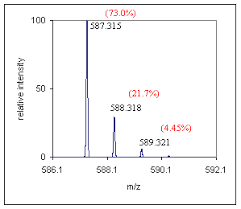The image above represents the mass of an element.

To compute for the mass of an element, two essential parameters are needed and these parameters are Electrochemical Equivalence of the Substance (Z) and quantity of charge (Q).

The formula for calculating mass of an element:

M = ZQ

Where;
M = Mass of the element
Z = Electrochemical Equivalence of the Substance
Q = Quantity of Charge

Let’s solve an example;
Find the mass of an element when the Quantity of charge is 28 and Electrochemical Equivalence of the Substance is 32.

This implies that;
Z = Electrochemical Equivalence of the Substance = 32
Q = Quantity of Charge = 28

M = ZQ
M = 32 x 28
M = 896

Therefore, the mass of an element is 896 kg.

Calculating the Electrochemical Equivalence of the Substance when the Mass of an Element and Quantity of Charge is Given.

Z = M / Q

Where;
Z = Electrochemical Equivalence of the Substance
M = Mass of the element
Q = Quantity of Charge

Let’s solve an example;
Find the Electrochemical Equivalence of the Substance when the Quantity of charge is 12 and  mass of an element  is 120.

This implies that;
M = Mass of the element = 120
Q = Quantity of Charge = 12

Z = M / Q
Z = 120 / 12
Z = 10

Therefore, the Electrochemical Equivalence of the Substance is 10.

Calculating the Quantity of Charge when the Mass of an Element and Electrochemical Equivalence of the Substance is Given.

Q = M / Z

Where;
Q = Quantity of Charge
M = Mass of the element
Z = Electrochemical Equivalence of the Substance

Let’s solve an example;
Find the Quantity of charge when the Electrochemical Equivalence of the Substance is 20 and  mass of an element  is 240.

This implies that;
M = Mass of the element = 240
Z = Electrochemical Equivalence of the Substance = 20

Q = M / Z
Q = 240 / 20
Q = 12

Therefore, the quantity of charge is 12 Coulomb (C).

Nickzom Calculator – The Calculator Encyclopedia is capable of calculating the mass of an element.

To get the answer and workings of the mass of an element using the Nickzom Calculator – The Calculator Encyclopedia. First, you need to obtain the app.

You can get this app via any of these means:

To get access to the professional version via web, you need to register and subscribe for NGN 1,500 per annum to have utter access to all functionalities.
You can also try the demo version via https://www.nickzom.org/calculator

Once, you have obtained the calculator encyclopedia app, proceed to the Calculator Map, then click on Electrolysis under Physics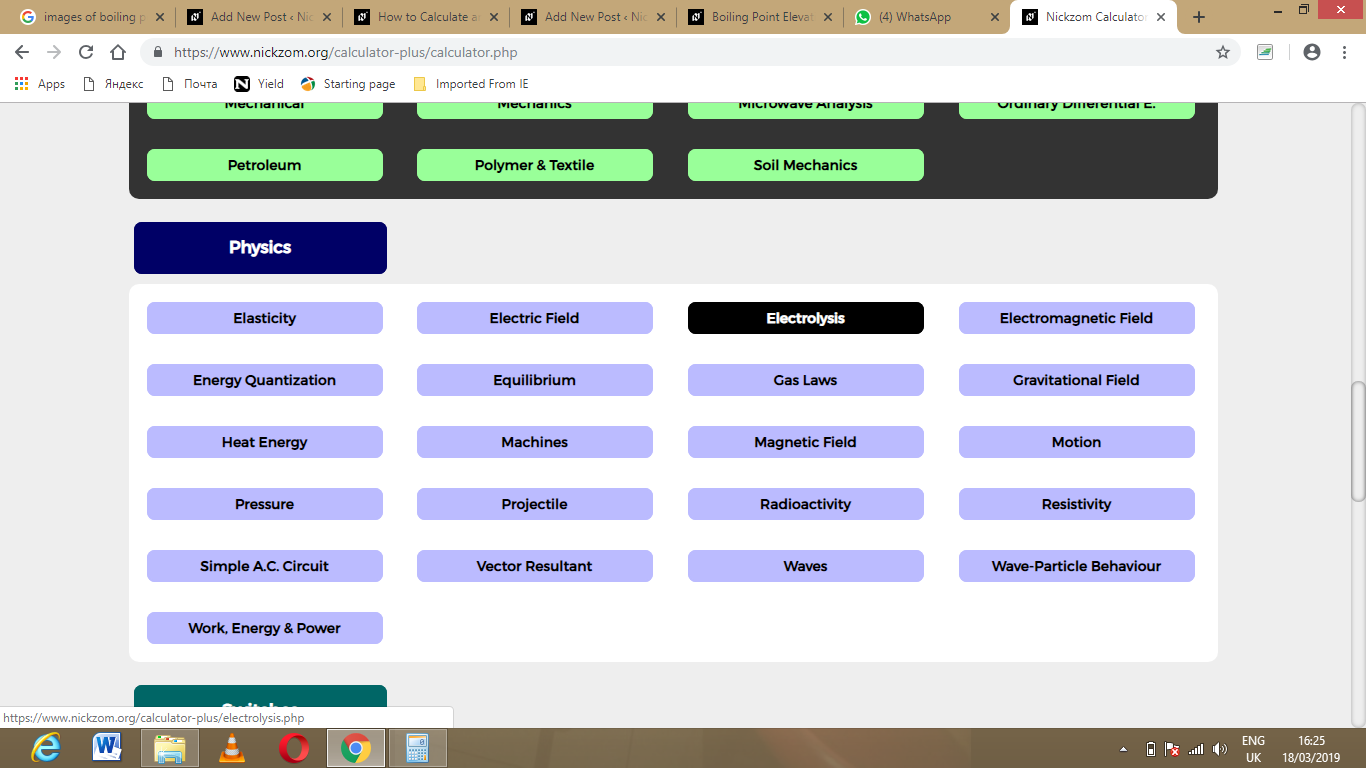Now, Click on Mass of an Element under Electrolysis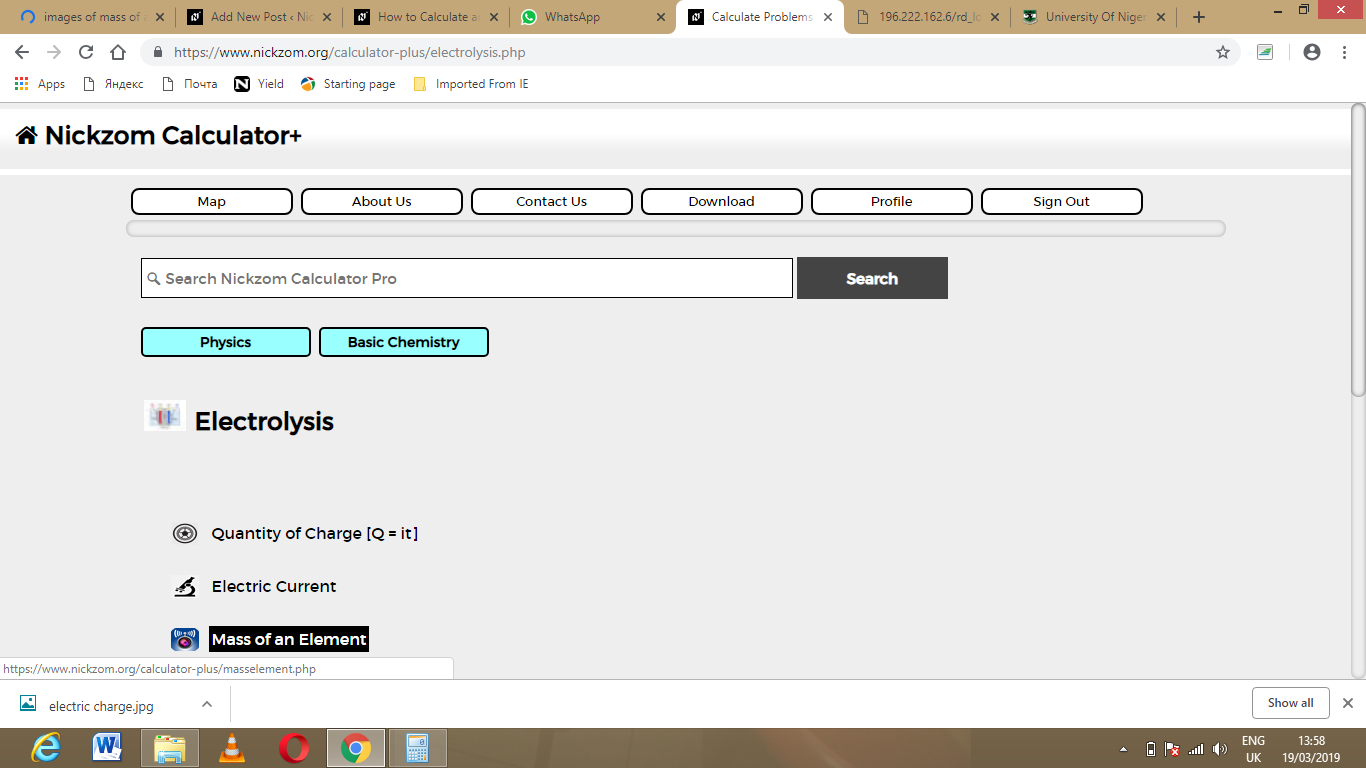The screenshot below displays the page or activity to enter your values, to get the answer for the mass of an element according to the respective parameters which are the electrochemical equivalence of the substance (Z) and Quantity of charge (Q).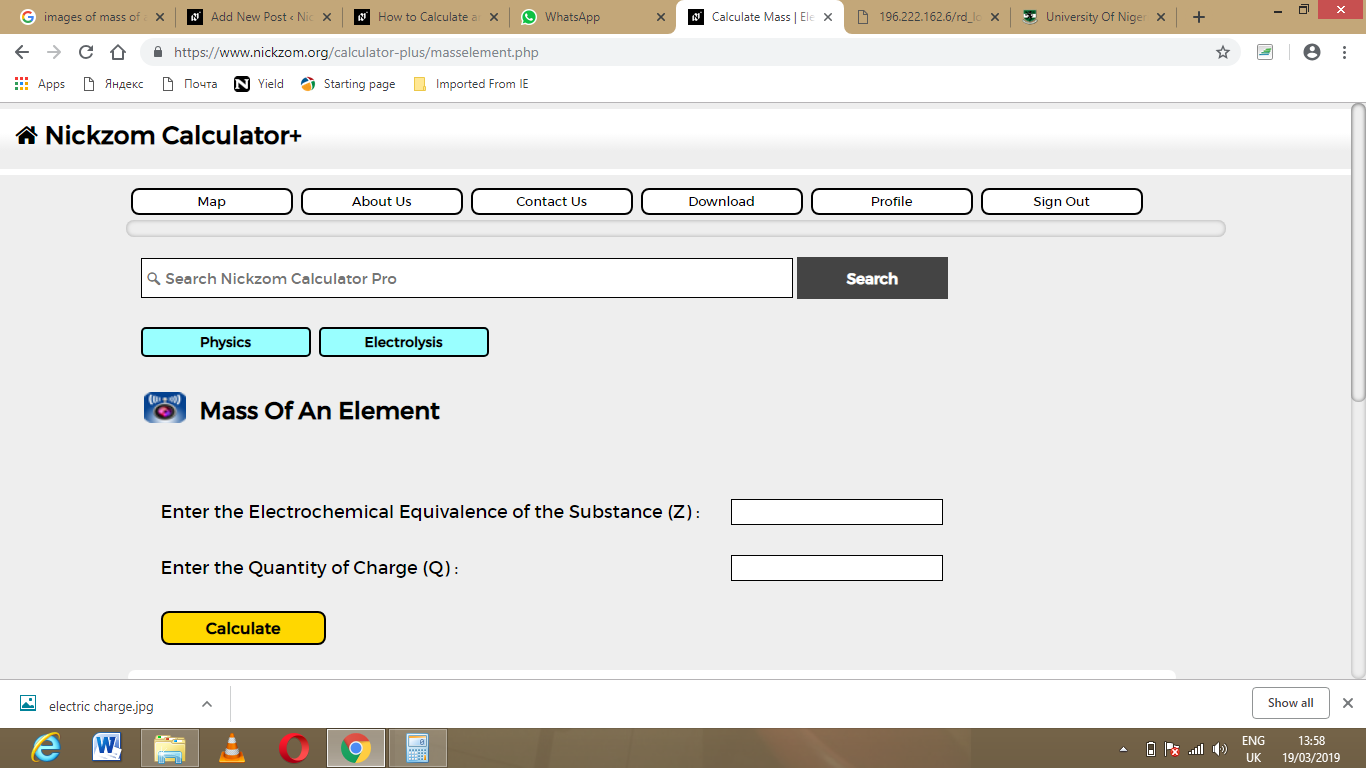Now, enter the values appropriately and accordingly for the parameters as required by the example above where the electrochemical equivalence of the substance (Z) is 32 and Quantity of charge (Q) is 28.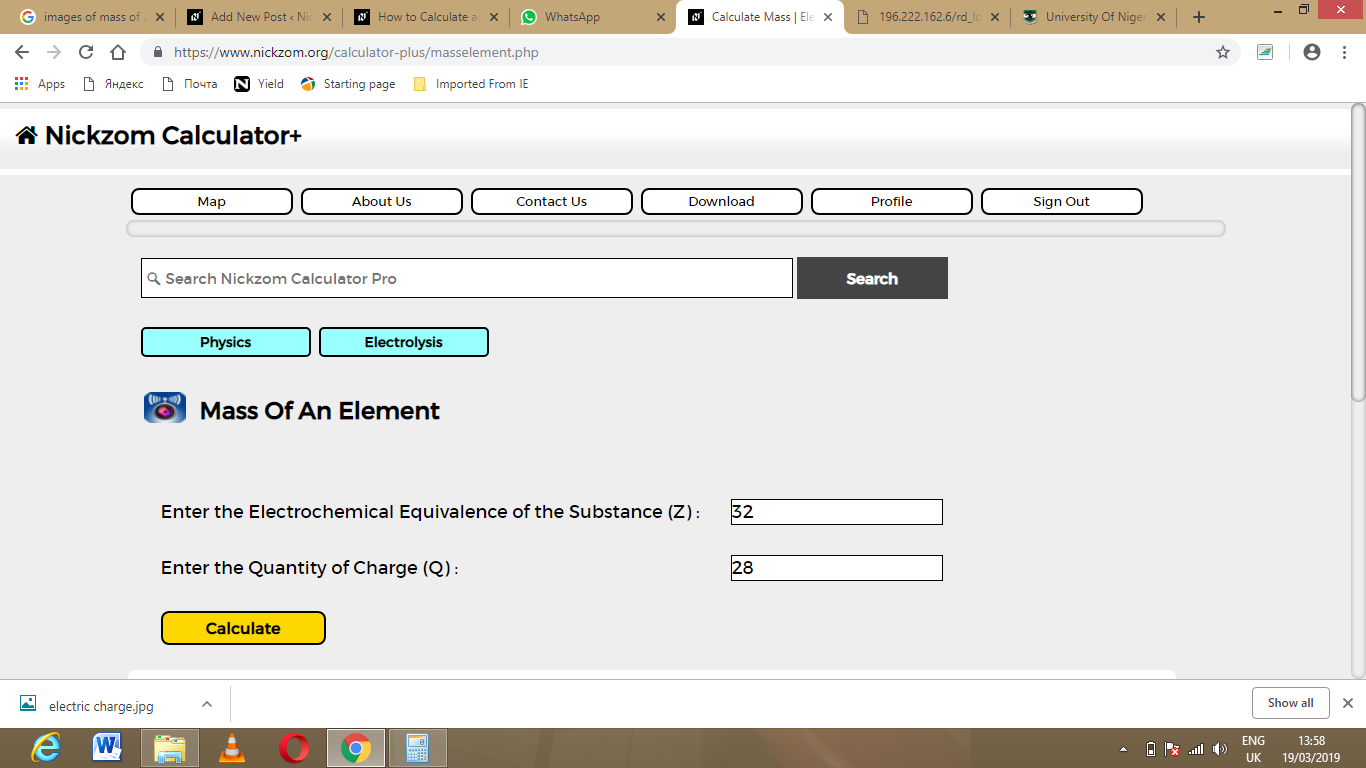Finally, Click on Calculate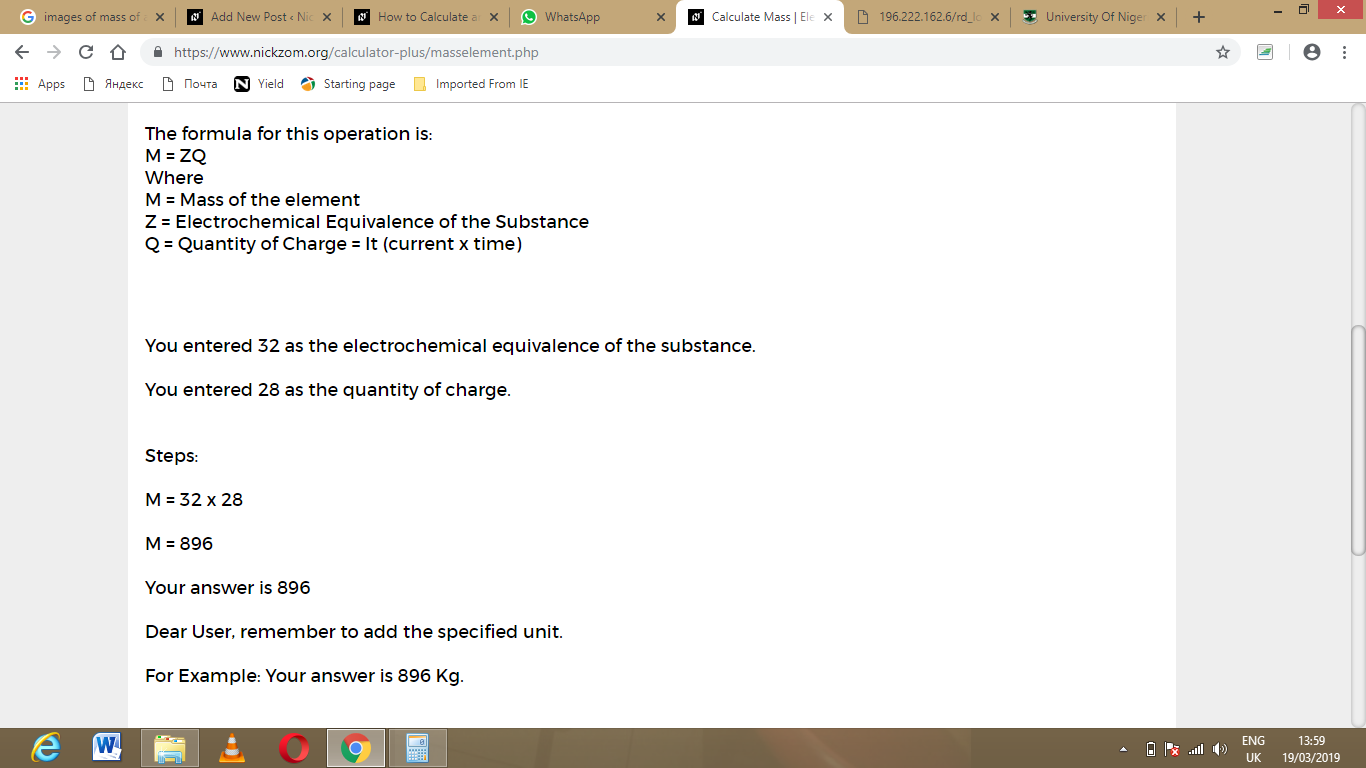As you can see from the screenshot above, Nickzom Calculator – The Calculator Encyclopedia solves for the mass of an element and presents the formula, workings and steps too.